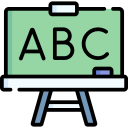# Problem Set #8: Context-Free Grammars

### Objective

During this activity, students should be able to:

• Solve problems that involve designing context-free grammars (CGF).

This activity helps students develop the following skills, values and attitudes: ability to analyze and synthesize, capacity for identifying and solving problems, and efficient use of computer systems.

## Activity Description

Solve with your team the exercises described in this problem set.

For each problem design the context-free grammar that accepts the described language and code the corresponding parser using the instaparse Clojure library. Make sure it passes all the unit tests.

Create a namespace called grammar. At the begining of the file add the following code in order to declare the namespace, import the required external functions, and define a couple of auxilary functions:

(ns grammar
(:require [clojure.test :refer [deftest is run-tests]])
(:require [instaparse.core :refer [parser]])
(:import (instaparse.gll Failure)))

(defn fails? [r] (instance? Failure r))
(defn succeeds? [r] (not (fails? r)))


(run-tests)

All the code you write should go between these two instructions.

1. A language with alphabet $$\Sigma = \{ \texttt{#}, \texttt{\} \}$$ that accepts all nonempty strings that start and end with the same symbol.

(def start-and-end (parser ""))


Unit tests:

(deftest test-start-and-end
(is (succeeds? (start-and-end "$"))) (is (succeeds? (start-and-end "#"))) (is (succeeds? (start-and-end "$$"))) (is (succeeds? (start-and-end "##"))) (is (succeeds? (start-and-end "$$$#$$#$$#$##$")))
(is (succeeds? (start-and-end "#$$#$$$$#$$$$#####"))) (is (fails? (start-and-end ""))) (is (fails? (start-and-end "#"))) (is (fails? (start-and-end "#"))) (is (fails? (start-and-end "###$$#$#$$#$$$####$"))) (is (fails? (start-and-end "$#$#$##$$#$$$$#$$#")))
(is (fails? (start-and-end "#######################\$"))))

2. $$\{w \in \{ 0, 1 \}^*\; | \;w = w^R \}$$. $$w^R$$ is the reverse of $$w$$. This language accepts a possibly empty sequence of zeros and ones that happens to be a palindrome.

;;; Parser:
(def palindrome (parser ""))


Unit tests:

(deftest test-palindrome
(is (succeeds? (palindrome "")))
(is (succeeds? (palindrome "0")))
(is (succeeds? (palindrome "1")))
(is (succeeds? (palindrome "11")))
(is (succeeds? (palindrome "00")))
(is (succeeds? (palindrome "010")))
(is (succeeds? (palindrome "1111111")))
(is (succeeds? (palindrome "000010000")))
(is (succeeds? (palindrome "01001110101110010")))
(is (fails? (palindrome "01")))
(is (fails? (palindrome "10")))
(is (fails? (palindrome "1010")))
(is (fails? (palindrome "10000000")))
(is (fails? (palindrome "00010001")))
(is (fails? (palindrome "1010011010")))
(is (fails? (palindrome "111111111111111111110"))))

3. A language with alphabet $$\Sigma = \{ \texttt{(}, \texttt{)} \}$$ that accepts all strings containing balanced parentheses. A string of parentheses is balanced if each left parenthesis has a matching right parenthesis and the matched pairs are well nested.

(def balanced-parentheses (parser ""))


Unit tests:

(deftest test-balanced-parentheses
(is (succeeds? (balanced-parentheses "")))
(is (succeeds? (balanced-parentheses "()")))
(is (succeeds? (balanced-parentheses "((((()))))")))
(is (succeeds? (balanced-parentheses "()()()()()")))
(is (succeeds? (balanced-parentheses
"(()()())((())(()()()))(((())))")))
(is (fails? (balanced-parentheses "(")))
(is (fails? (balanced-parentheses ")")))
(is (fails? (balanced-parentheses "((((())))")))
(is (fails? (balanced-parentheses "))))((((")))
(is (fails? (balanced-parentheses
"(()()())((())(()()()))((((())))"))))

4. A language with alphabet $$\Sigma = \{ o, x \}$$ that accepts all strings with an odd length and $$o$$ as its symbol in the middle.

(def o-in-the-middle (parser ""))


Unit tests:

(deftest test-o-in-the-middle
(is (succeeds? (o-in-the-middle "o")))
(is (succeeds? (o-in-the-middle "xox")))
(is (succeeds? (o-in-the-middle "oooooxxxx")))
(is (succeeds? (o-in-the-middle "oxxoooxooxoxoxx")))
(is (succeeds? (o-in-the-middle "ooooooooooooooo")))
(is (succeeds? (o-in-the-middle "xxxxxxxxxxoxxxxxxxxxx")))
(is (fails? (o-in-the-middle "")))
(is (fails? (o-in-the-middle "ox")))
(is (fails? (o-in-the-middle "oxo")))
(is (fails? (o-in-the-middle "oxxoooxxoxoxoxx")))
(is (fails? (o-in-the-middle "xxxxxxxxxxxxxxxxxxxxx")))
(is (fails? (o-in-the-middle "oooooooooooooooooooooo"))))

5. $$\{x^{2n}y^n\; | \;n \ge 1 \}$$. This language accepts a nonempty sequence of consecutive symbols $$x$$ followed by a nonempty sequence of consecutive symbols $$y$$. The number of symbols $$x$$ has to be exactly twice the number of symbols $$y$$.

(def twice (parser ""))


Unit tests:

(deftest test-twice
(is (succeeds? (twice "xxy")))
(is (succeeds? (twice "xxxxyy")))
(is (succeeds? (twice "xxxxxxxxyyyy")))
(is (succeeds? (twice "xxxxxxxxxxxxxxxxxxxxyyyyyyyyyy")))
(is (fails? (twice "")))
(is (fails? (twice "xy")))
(is (fails? (twice "yxx")))
(is (fails? (twice "xyy")))
(is (fails? (twice "xxxyyy")))
(is (fails? (twice "xyyxyy")))
(is (fails? (twice "xxxxyyx")))
(is (fails? (twice "yyyxxxxxx")))
(is (fails? (twice "xxxxxxxxxxyy")))
(is (fails? (twice "xyxyxyxyxxxx")))
(is (fails? (twice "xxxxxxxxxxyyyy"))))

6. $$\{ a^i b^j c^k\; | \;i, j, k \ge 0 \textrm{ and } i + j = k \}$$. This language accepts a possibly empty sequence of consecutive symbols $$a$$ followed by a possibly empty sequence of consecutive symbols $$b$$ followed by a possibly empty sequence of consecutive symbols $$c$$. The number of symbols $$c$$ has to be exactly the number symbols $$a$$ plus the number symbols $$b$$.

(def a-plus-b-equals-c (parser ""))


Unit tests:

(deftest test-a-plus-b-equals-c
(is (succeeds? (a-plus-b-equals-c "")))
(is (succeeds? (a-plus-b-equals-c "abcc")))
(is (succeeds? (a-plus-b-equals-c "ac")))
(is (succeeds? (a-plus-b-equals-c "bc")))
(is (succeeds? (a-plus-b-equals-c "aaaaabbbcccccccc")))
(is (succeeds? (a-plus-b-equals-c "aaaaaaaaabcccccccccc")))
(is (succeeds? (a-plus-b-equals-c
"bbbbbbbbbbbbbccccccccccccc")))
(is (succeeds? (a-plus-b-equals-c
"abbbbbbbbbbbbccccccccccccc")))
(is (succeeds? (a-plus-b-equals-c
"aaaabbbbbbbbbbbccccccccccccccc")))
(is (fails? (a-plus-b-equals-c "a")))
(is (fails? (a-plus-b-equals-c "b")))
(is (fails? (a-plus-b-equals-c "c")))
(is (fails? (a-plus-b-equals-c "ab")))
(is (fails? (a-plus-b-equals-c "abc")))
(is (fails? (a-plus-b-equals-c "abbcc")))
(is (fails? (a-plus-b-equals-c "cccccc")))
(is (fails? (a-plus-b-equals-c "ccccaabb")))
(is (fails? (a-plus-b-equals-c "aaaaaaaa")))
(is (fails? (a-plus-b-equals-c "bbbbbbbbb")))
(is (fails? (a-plus-b-equals-c "aaaaaabbbbbb")))
(is (fails? (a-plus-b-equals-c "aabbcccccccccccccccccc"))))


## Deliverables

The program source file must include at the top the authors’ personal information (student ID and name) within comments. For example:

;----------------------------------------------------------
; Problem Set #8: Context-Free Grammars
; Date: May 26, 2023.
; Authors:
;          A01770771 Sylvie Laufeydottir
;          A01777771 Loki Laufeyson
;----------------------------------------------------------


### Instrucciones para subir archivo

Para entregar el archivo grammar.clj, ingresa los siguientes datos:

Only one team member needs to upload the file.

Due date is Friday, May 26.

## Evaluation

This activity will be evaluated using the following criteria:

 -10 The program doesn't contain within comments the author’s personal information. The program contains syntax errors. The program was plagiarized in whole or in part. Depending on the amount of exercises that were solved correctly.